# Geometry - math word problems

1. MO Z9–I–2 - 2017In the VODY trapezoid, VO is a longer base and the diagonal intersection K divides the VD line in a 3:2 ratio. The area of the KOV triangle is 13.5 cm2. Find the area of the entire trapezoid.
2. Points on line segmentPoints P & Q belong to segment AB. If AB=a, AP = 2PQ = 2QB, find the distance: between point A and the midpoint of the segment QB.
3. Right angled triangle 2LMN is a right angled triangle with vertices at L(1,3), M(3,5) and N(6,n). Given angle LMN is 90° find n
4. Line segmentCut a line segment of 15 cm into two line segments so that their lengths are in ratio 2:1. What length will each have?
5. LinesHow many lines can be draw with 8 points, if three points lie on one line and the other any three points do not lie on the same line?
6. Parcel 4To send a parcel by messenger within city limits costs 60 cents for the first pound and 48 cents for each additional pound. What is the cost, in cents, of sending a parcel weighing p=4 pounds?
7. GarageThere are two laths in the garage opposite one another: one 2 meters long and the second 3 meters long. They fall against each other and stay against the opposite walls of the garage and both laths cross 70 cm above the garage floor. How wide is the gara
8. Vector 7Given vector OA(12,16) and vector OB(4,1). Find vector AB and vector |A|.
9. Similarity coefficient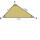The triangles ABC and A "B" C "are similar to the similarity coefficient 2. The sizes of the angles of the triangle ABC are α = 35° and β = 48°. Find the magnitudes of all angles of triangle A "B" C ".
10. Mirror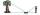How far must Paul place a mirror to see the top of the tower 12 m high? The height of Paul's eyes above the horizontal plane is 160 cm and Paul is from the tower distant 20 m.
11. ThalesThales is 1 m from the hole. The eyes are 150 cm above the ground and look into the hole with a diameter of 120 cm as shown. Calculate the depth of the hole.
12. Display case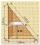Place a glass shelf at the height of 1m from the bottom of the display case in the cabinet. How long platter will we place at this height? The display case is a rectangular triangle with 2 m and 2.5 m legs.
13. Rhombus EFGHConstruct the rhombus EFGH where e = 6.7cm, height to side h: vh = 5cm
14. Hexagon cut pyramidCalculate the volume of a regular 6-sided cut pyramid if the bottom edge is 30 cm, the top edge us 12 cm, and the side edge length is 41 cm.
15. Linear function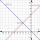What is the equation of linear function passing through points: a) A (0,3), B (3,0) b) A (-2,-6), B (3,4)
16. Vertices of RTShow that the points P1 (5,0), P2 (2,1) & P3 (4,7) are the vertices of a right triangle.Show that the quadrilateral with vertices P1(0,1), P2(4,2) P3(3,6) P4(-5,4) has two right triangles.
18. Forces on earth directionsA force of 60 N [North] and 80 N [East] is exerted on an object wigth 10 kg. What is the acceleration of the object?
19. Sides od triangleSides of the triangle ABC has length 4 cm, 5 cm and 7 cm. Construct triangle A'B'C' that are similar to triangle ABC which has a circumference of 12 cm.
20. V-belt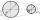Calculate a length of the V-belt when the diameter of the pulleys is: D1 = 600 mm D2 = 120 mm d = 480 mm

Do you have an interesting mathematical word problem that you can't solve it? Submit math problem, and we can try to solve it.

We will send a solution to your e-mail address. Solved examples are also published here. Please enter the e-mail correctly and check whether you don't have a full mailbox.

Please do not submit problems from current active competitions such as Mathematical Olympiad, correspondence seminars etc...